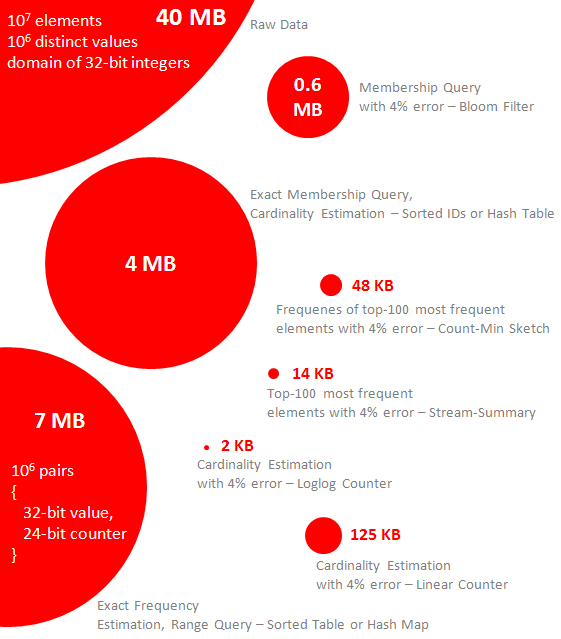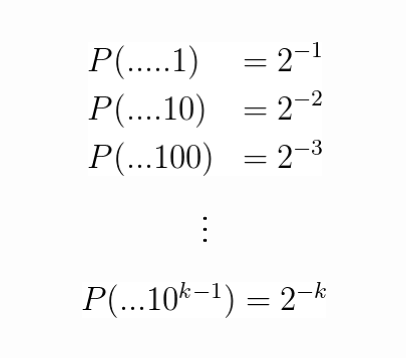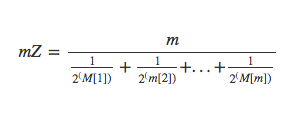# Curiosity #2: How does Prestodb implement approx_distinct?

This article is part of the curiosity series in which I will document and share my learnings guided by random curiosity. The purpose is to share knowledge as well as enhance my understanding through teaching. Read Curiosity #1: Sound.

### Context:

What is Prestodb?

Prestodb is an open source query engine which provides a ANSI SQL query language. It runs its’ queries in memory. https://prestodb.io/

What is approx_distinct?

It is an aggregate function which approximates count(distinct x). See the documentation here: https://prestodb.io/docs/current/functions/aggregate.html#approx_distinct

approx_distinct is not available in MySQL, which made me very curious to know how is it implemented. It has computational efficiencies compared to count(distinct x).

How does approx_distinct approximate the number of distinct values?

Luckily, Prestodb is open source, and I was able to find the code for approx_distinct here:

And found out it depended on this import for its functionality:

import io.airlift.stats.cardinality.HyperLogLog;

At this point I didn’t know what HyperLogLog (HLL) was. But when I reached this piece of documentation for it in the airlift library, I figured it was probably a common thingy (I say thingy, because at this point I have no clue what it is):

What is HyperLogLog?

HyperLogLog (HLL) is a probabilistic data structure which approximates the cardinality (number of distinct values) in a stream of data. This means that HLL can be used in stream processing too.

What is a probabilistic data structure?

A probabilistic data structure is one which trades accuracy of information for lesser space complexity and/or lesser time complexity using statistical properties of the data.Figure 1: common probabilistic data structures with errors and memory requirements [source]

The following article goes into brief detail on different bloom filters, HLL, and a couple other probabilistic data structures. Please read it if you want to understand bloom filters:

Here’s another introduction to these probabilistic data structures:

Okay back to HLL! After reading and watching the above linked content, I had a basic vague idea of what HLL was and how it worked. But my goal was understanding, thus I dove in an read the paper for HLL: http://algo.inria.fr/flajolet/Publications/FlFuGaMe07.pdf

No worries if you don’t want to read through that, just keep reading below.

### How the HyperLogLog algorithm works:

Please read the HyperLogLog section in this link before continuing: http://bravenewgeek.com/stream-processing-and-probabilistic-methods/

As the rest of this section will be a deep dive into the implementation and a bit into the statistics of how HLL works.

HLL uses the probability of consecutive 0’s appearing in a binary string. The binary string is the result of hashing the value. A good hashing function should have results uniformly distributed, meaning that the probability of any one result occurring should be equal to any other result occurring. It’s this property of hashing functions that most probabilistic data structures take advantage of.

The probability of consecutive 0’s appearing in a binary string can be rephrased as the probability of the left most 1 bit being at position x, where x is a positive integer. Let’s call this p(x).

p(1) is 1/2 because there can only be two possible values in position 1, which is 0 or 1. Thus the probability that it is a 1 is 1/2.

p(2) is 1/4 because there can be 4 different values for the first two positions: 00, 01, 10, 11. 1/4 of those values have the left most 1 bit at position 2 (value 01), thus this is the probability.

Extending that we get:Figure 2: p(x) — Probability that left most 1 bit is in position x [source]

To reduce the error on HLL multiple “experiments” are completed. This is done by splitting the input stream into multiple streams — each value in the input stream will go to one of m streams. The values are sent to a stream based on their hashed value, which makes it pseudo-random which stream it goes to. These experiments are taking advantage of statistical experimentation properties. It is similar to taking a sample population of N (total values in the stream) / m for each of the m experiments.

Each “experiment” will keep track of x (the largest position of the left most 1 bit), and the harmonic mean of p(x) of the experiments is used to determine the expected value for cardinality. The harmonic mean is the reciprocal of the arithmetic mean (which is the average we are used to). The arithmetic mean skews higher when a high value is part of it’s input set, but since the harmonic mean is the reciprocal it skews lower when a low value is part of it’s input set. For example, the arithmetic mean of 1, 2, 3, 100 is 26.5 and the harmonic mean is 2.17. And the arithmetic mean of 100, 101, 102, 1 is 76 and the harmonic mean is 3.88.

Let’s dig into how the algorithm is ran step by step and what computations in the HLL algorithm are.

There will be m number of experiments, where m must be a power of 2. For each experiment, its register holds the largest position of the left most 1-bit.

For example, let’s pick b = 5 and m = 2⁵ = 32.

For every value, v, in the stream of values:

• the hash value, x, is calculated
• j is determined by looking at the first b bits of the hashed value, x, and tells us which experiment this value will be part of.
• w is the position of the left most 1 bit starting from the bits after b
• if w is bigger than the value in the register for experiment j (aka previously calculated largest position of left most 1 bit), the register is updated.

Let’s use the hashing function used by Prestodb’s approx_distinct, Murmur3–128 for our example. This hashing function returns a 128 bit value.

Given v = “Hello World”

x = 1a6326abc1a0c2db83e61fcf9fc0b427 [using online hashing tool]

The binary value of x is 00110001 01100001 00110110 00110011 00110010 00110110 01100001 01100010 01100011 00110001 01100001 00110000 01100011 00110010 01100100 01100010 00111000 00110011 01100101 00110110 00110001 01100110 01100011 01100110 00111001 01100110 01100011 00110000 01100010 00110100 00110010 00110111 [using online converter]

Looking at the first b bits, recall we are using b = 5, we have 00110, we convert this back to a decimal number.

j = 1 + 00110b

j = 1 + 6 [using online converter]

j = 7

This means that “Hello World” was assigned to be part of the 7th experiment.

x in binary is 00110001 01100001…. to calculate w, the bits used for j is disregarded, only look at w = …001 01100001….

Looking at the bits after the 5th bit, the position left most 1 bit relative to the 5th bit is 3.

p(w) = 3

Important note! above we used p(x) is the probability that the left most 1 bit is in position x. Here p(w) is the position of the left most 1 bit.

Use this demo to explore more example hash values and there derived values for HLL: https://research.neustar.biz/2013/04/02/sketch-of-the-day-probabilistic-counting-with-stochastic-averaging-pcsa/ *Note that this demo uses a different hashing function than we have used in our example above.

Once every value in the stream is evaluated, the “indicator” function and the expected cardinality are calculated.

Recall that the probability of the left most 1 bit being at a position is p(x) = 2^-x where x is the position. Thus Z is 1 over the sum of the probabilities for each experiment m, using the largest position of the left most 1 bit from that experiment.

Rewriting these equations out, mZ is the harmonic mean of the probabilities.Left: Harmonic mean equation, Right: mZ rewritten

To get E, the expected cardinality, the harmonic mean is multiplied by the number of experiments, m. Note that alpha here is the statistical alpha, read more about it here.

Awesome, we now know how HLL works internally using probability of consecutive values (in this case 0’s) and separating the stream into experiments to decrease error.

HLL also does corrections when the range of values in the stream is small and when it’s very large to decrease error. But actually the use of the harmonic mean itself also decreases the error…

### How accurate is HyperLogLog?

“HYPERLOGLOG performs a single pass over the data and produces an estimate of the cardinality such that the relative accuracy (the standard error) is typically about 1.04/ √ m. This improves on the best previously known cardinality estimator, LOGLOG, whose accuracy can be matched by consuming only 64% of the original memory. For instance, the new algorithm makes it possible to estimate cardinalities well beyond 10^9 with a typical accuracy of 2% while using a memory of only 1.5 kilobytes.” — from the abstract of the HLL paper

The difference in HLL and LogLog (LL), it’s predecessor is that LL uses the arithmetic mean and HLL uses the harmonic mean. It is through the properties of the different means that the standard error for a given number of experiments m is reduced. Thus to get the same standard error, HLL uses less experiments thus less registers/memory. This is why HLL needs only 64% of the memory that LL needs to achieve the same standard error.

### The Curiosity Series

This article is part of the curiosity series in which I will document and share my learnings guided by random curiosity. The purpose is to share knowledge as well as enhance my understanding through teaching.

Articles will consist more or less of links to information for you to explore as well as some of my notes and summaries. The overarching goal is for us to become more curious individuals, to gain the confidence in our learning capabilities, and to grow our growth mindset.

### Call To Action

Now that you’ve learnt about HyperLogLog and how it works in detail, I highly encourage you to pick a topic and explore further! Here’s a list of suggestions:

• How are the registers actually stored in approx_distinct?
• How does Prestodb implement approx_percentile?
• What are some other probabilistic data structures, and what are the trades off with HLL? When would you want to use one versus the other?
• In this hacker news: https://news.ycombinator.com/item?id=6684678, approximate queries was mentioned, how can approximate queries be implemented?

And if you enjoyed this article please give it a clap and subscribe to see more articles like this in the future.